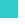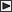BRI Research Paper

 No.62 Numerical Calculation of Room Air Movement -Isothermal Turbulent Two-Dimensional Case-. T.Tsuchiya; January, 1976. 24p. Abstract This paper presents a numerical calculation method for a two-dimensional,isothermal, turbulent room air movement. In this case, the time averaged stream function-vorticity equations were represented by finite differencing approximations with a box model and a leapfrog time scheme and the Reynolds stresses were assumed to be expressed in terms of an eddy kinematic viscosity which was estimated to be proportional to the product of the cube of a prescribed mixing length by a vorticity gradient. This proportional constant was determined by comparing the computed velocity distributions with experiments for two basic flows. Furthermore, the parameterizations were proposed concerning the boundary conditions of vorticity on a wall which corrected the gaps between the true velocity and vorticity gradients and the represented by a finite differencing. These parameters were also determined as compared with experiments. Then, three obtained parameters were confirmed to be valid by applying to a rather complicated flow in which an air curtain was affected by a side blow. ** Chief,Environmental Design Sect., Building Research Institute, Ministry of ConstructionLinksSitemapInquiryJapanese HP

BUILDING RESEARCH INSTITUTE, JAPAN